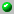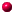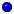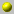the following papers are available on our server= dvi format= ps format= tex format= pdf formata_character_formula_for_a_family_of_simple_modular_representations_of_GL_na_character_formula_for_a_family_of_simple_modular_representations_of_GL_na_combinatorial_character_formula_for_some_highest_weight_modulesa_combinatorial_character_formula_for_some_highest_weight_modulescan_fusion_coefficients_be_calculated_from_the_depth_rulecan_fusion_coefficients_be_calculated_from_the_depth_rulecan_fusion_coefficients_be_calculated_from_the_depth_ruleclassification_of_irreducible_weight_modulesclassification_of_irreducible_weight_modules

Go back to the directory of pre and reprints.
Or search the database by keywords
Or look for the newest entires in the database